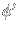Billiards

## Birkhoff billiardsBIRKHOFF BILLIARDS: The motion of a free particle in a bounded region reflecting elastically at the boundary is called a billiard . Convex two-dimensional convex regions in the plane define Birkhoff billiards . A Birkhoff billiard is smooth if the boundary of the table is described by arbitrarily often differentiable functions. EXAMPLES: 1) Ellipse: x2/a2 + y2/b2 = 1. 2) Polygons: eg. triangle 3) Polygons with rounded corners: eg. stadium 4) Tables of equal width: eg. Ruleaux triangle
 BILLIARD PIONEERS:Boltzmann (1844-1906) hard sphere gas Artin (1898-1962) in 1924, billiard in hyperbolic plane Hadamard (1865-1963)-Hedlund-Hopf, geodesic flow Birkhoff (1884-1944) in 1927, model for 3-body problem Poritski in 1950, integrability question
WHY STUDY BILLIARDS?
Billiards ard beautiful and simple dynamical systems featuring many complexities of Hamiltonian systems in general. They form a limiting case of the geodesic flow and illustrate theorems in topology, geometry or ergodic theory. Some dynamical problems are related to the Dirichlet problemwhich still features Kac's problem "can one hear the shape of a smooth drum" (until now, known isospectral counterexamples to Kac's question are not smooth). Billiards are used to investigate the transition from quantum mechanics (the Dirichlet problem, asymptotics of eigenvalues and eigenfunctions) to classical mechanics (the geodesic flow).

## The map

 THE BILLIARD MAP: Let s be T = R (mod 1) a point on the boundary of the table and let: be the angle of the velocity vector to the tangent at s. Define. If s' is the new intersection with boundary andis defined from the impact angle. the billiard map on the annulus X = T x [-1,1] is The boundaries,consist of fixed points.

## Integrability and Chaos

 DEFINITION: INTEGRABILITY: A billiard map T:X -> X is called integrable if there exists a piecewise continuous f: X -> R such that each set {f(s,u)= c} is a finite union of discrete points or one-dimensional curves. (Warning: this is one of many definitions of integrability for billiards). DEFINITION: LYAPUNOV EXPONENT.is called the Lyapunov exponent of T at (s,u). It measures sensitive dependence on initial conditions. DEFINITION: CHAOS. A billiard is called chaotic if the Pesin sethas positive Lebesgue measure. No smooth, convex chaotic billiard is known! (Warning: the word "chaos" is used in many different ways).

## Area preservation

 The return map of a Birkhoff billiard is an area-preserving map on the annulus. Birkhof billiards become so a natural object in ergodic theory . By a change of variable formula this is equivalent to: Jacobiansatisfies.

## Periodic orbits

 Smooth strictly convex billiards have many periodic orbits: a ball which returns after p reflections back having been winding around q times around the table. These Birkhoff periodic orbits can be used to construct invariant continua of the map, so called Mather sets In some cases, these sets are Cantor sets, sometimes invariant curves.are points on, let, where. A periodic orbit Tk(s,u) =(sk, uk) corresponds to a critical point of, a point, where the gradientvanishes. There exists a maximum and so a critical point.

## Caustics

 DEFINITION: CAUSTICS: Curves for which tangent billiard trajectories remains tangent after successive reflections. EXAMPLES OF CAUSTICS 1) Ellipses have conformal conicsas caustics, hyperbola for, ellipses for. 2)convex curve, the string construction leads to table withas caustic. 3) Curves of equal width have caustics which agree with the evolute of the table.

## Invariant curves and Caustics

 INVARIANT CURVES-CAUSTICS. Ifis an invariant curve under T and v(s) is a unit vector in the trajectory direction, P(s) point on table,curvature at s, we get a caustic(Douady). PROOF. T(s,u) = (s1,u1)., angle of trajectory L(s,s1) to x-axes., where.: angle of tangent at T(s), then. Furthermore, so that.

## Curves of equal width

 CURVES OF EQUAL WIDTH.angle of tangent.radius of curvature at.defines a closed convex curve if. If additionally, this is a table of equal width. CAUSTIC AND EVOLUTE:defines caustic to invariant curve. This is the evolute of the curve.showsandhave identical critical points. Vertices of tablecusps of.

## Are there fractal caustics

 ARE THERE FRACTAL CAUSTICS? Are there caustics which are fractals? Are there fractal evolutes of convex curves of equal width? DEFINITION: FRACTALS. Z subset of an Euclidean space. For, define, whereruns over all open-covers of Z. (, Uj open and). The limitis called s-dimensional Hausdorff measure of Z. It exists inbecauseincreases for. If, then ht(Z)=0 for all t>s. Define the Hausdorff dimensionby,. Fractals are sets Z with non-integer.

## Some unsolved problems

 BIRKHOFF-PORITSKY CONJECTURE: if a smooth Birkhoff billiard is integrable then it is an ellipse. This is a bundle of many problems, because it depends on the notion of integrability. CHAOTIC BILLIARD PROBLEM: there is a smooth Birkhoff billiard which has positive Lyapunov exponents on a set of positive measure. PERIODIC ORBITS IN POLYGONS: if the Birkhoff billiard is a polygon, there exists a periodic orbit. FRACTAL CAUSTIC PROBLEM: Is there a Birkhoff billiard with a caustic which is a fractal: a set with Hausdorff dimension between 1 and 2? ALGEBRAIC RETURN MAP PROBLEM: Assume a Birkhoff billiard is given by an algebraic map in some coordinates. Is the table an ellipse? GUILLEMIN PROBLEM: T1,T2 smooth Birkhoff billiard maps. If T1 = S T1 S-1 with a homeomorphism S, then the tables are similar. SIZE OF PERIODIC ORBITS: the set of n-periodic orbits of a smooth strictly convex Birkhoff billiard is nowhere dense for all n.

## SOME LITERATURE:

 G. Birkhoff, Acta Math, 50, 1927 Ya.G. Sinai, Introduction to ergodic theory, 1976 S. Tabachnikov. Billiards, 1995 D.V. Treshchev, V.V. Kozlov, Billiards, Transl. Math. Monog., 89

 © 1999, Oliver Knill , dynamical-systems.org